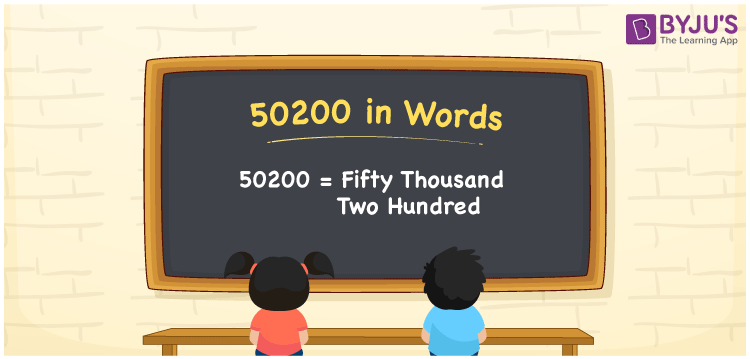# 50200 in Words

50200 in words is “fifty thousand two hundred”. For instance, Akshay contributed Rs. 50200 to an orphanage. It can also be written as “Akshay contributed Rs. Fifty thousand two hundred to an orphanage”. Also, the number 50200 is a cardinal number as it specifies the value of an object equivalent to fifty thousand two hundred.

 50200 in Words: Fifty Thousand Two Hundred. Fifty Thousand Two Hundred in Numerical Form: 50200.

## 50200 in English Words## How to Write 50200 in Words?

50200 is a natural number and its place values are presented below:

 Ten-thousands Thousands Hundreds Tens Ones 5 0 2 0 0

The expanded form of 50200 is as follows:

= 5 × Ten thousand + 0 × Thousand + 2 × Hundred + 0 × Ten + 0 × One

= 5 × 10000 + 0 × 1000 + 2 × 100 + 0 × 10 + 0 × 1

= 50000 + 200

= 50200

= Fifty thousand two hundred

Hence, 50200 in words is fifty thousand two hundred.

50200 in words – Fifty thousand two hundred

Is 50200 an odd number? – No

Is 50200 an even number? – Yes

Is 50200 a perfect square number? – No

Is 50200 a perfect cube number? – No

Is 50200 a prime number? – No

Is 50200 a composite number? – Yes

## Frequently Asked Questions on 50200 in Words

Q1

### Write 50200 in words.

50200 in words is fifty thousand two hundred.

Q2

### Simplify 50000 + 200, and express it in words.

Simplifying 50000 + 200, we get 50200. Hence, 50200 in words is fifty thousand two hundred.

Q3

### Is 50200 a composite number?

Yes, 50200 is a composite number.